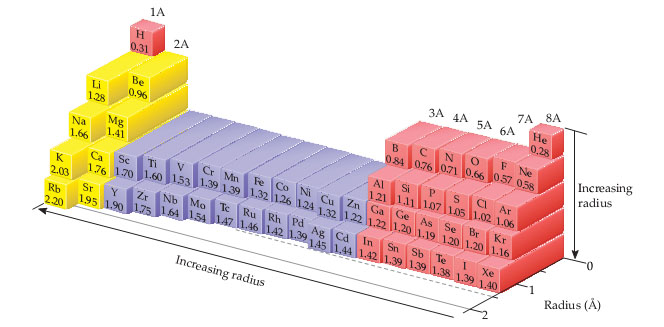# Problem: You may want to reference (Pages 263 - 267) Section 7.3 while completing this problem.Which of the following statements about the bonding atomic radii in the following figure is incorrect?(i) For a given period, the radii of the representative elements generally decrease from left to right across a period.(ii) The radii of the representative elements for the n = 3 period are all larger than those of the corresponding elements in the n = 2 period.(iii) For most of the representative elements, the change in radius from the n = 2 to the n = 3 period is greater than the change in radius from n = 3 to n = 4.(iv) The radii of the transition elements generally increase moving from left to right within a period.(v) The large radii of the Group 1A elements are due to their relatively small effective nuclear charges

###### FREE Expert Solution
83% (385 ratings)###### Problem Details

You may want to reference (Pages 263 - 267) Section 7.3 while completing this problem.

Which of the following statements about the bonding atomic radii in the following figure is incorrect?(i) For a given period, the radii of the representative elements generally decrease from left to right across a period.
(ii) The radii of the representative elements for the n = 3 period are all larger than those of the corresponding elements in the n = 2 period.
(iii) For most of the representative elements, the change in radius from the n = 2 to the n = 3 period is greater than the change in radius from n = 3 to n = 4.
(iv) The radii of the transition elements generally increase moving from left to right within a period.
(v) The large radii of the Group 1A elements are due to their relatively small effective nuclear charges

Frequently Asked Questions

What scientific concept do you need to know in order to solve this problem?

Our tutors have indicated that to solve this problem you will need to apply the Periodic Trend: Atomic Radius concept. You can view video lessons to learn Periodic Trend: Atomic Radius. Or if you need more Periodic Trend: Atomic Radius practice, you can also practice Periodic Trend: Atomic Radius practice problems.

What professor is this problem relevant for?

Based on our data, we think this problem is relevant for Professor Young's class at UCSD.